# Integral extension of a ring

(diff) ← Older revision | Latest revision (diff) | Newer revision → (diff)

An extensionof a commutative ringwith unit element such that every element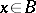is integral over, that is, satisfies an equation of the form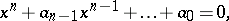where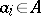, a so-called equation of integral dependence.

An elementis integral overif and only if one of the following two equivalent conditions is satisfied: 1)is an-module of finite type; or 2) there exists a faithful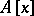-module that is an-module of finite type. An integral element is algebraic over. Ifis a field, the converse assertion holds. Elements of the fieldof complex numbers that are integral over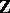are called algebraic integers. If a ringis a module of finite type over, then every elementis integral over(the converse need not be true).

Suppose thatis a commutative ring, and letandbe elements ofthat are integral over. Then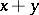and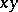are also integral over, and the set of all elements ofthat are integral overforms a subring, called the integral closure ofin. All rings considered below are assumed to be commutative.

Ifis integral overand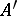is some-algebra, then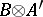is integral over. Ifis an integral extension ofandis some multiplicative subset of, then the ring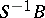is integral over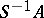. An integral domainis said to be integrally closed if the integral closure ofin its field of fractions is. A factorial ring is integrally closed. A ringis integrally closed if and only if for every maximal ideal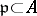the local ring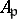is integrally closed.

Letbe an integral extension ofand letbe a prime ideal of. Then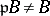and there exists a prime ideal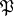ofthat lies above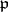(that is,is such that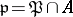). The idealis maximal if and only ifis maximal. Ifis a finite extension of the field of fractions of a ringandis the integral closure ofin, then there are only finitely-many prime ideals ofthat lie above a given prime ideal of.

Suppose that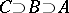; thenis an integral extension if and only if bothand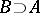are integral extensions.

How to Cite This Entry:
Integral extension of a ring. Encyclopedia of Mathematics. URL: http://encyclopediaofmath.org/index.php?title=Integral_extension_of_a_ring&oldid=17415
This article was adapted from an original article by L.V. Kuz'min (originator), which appeared in Encyclopedia of Mathematics - ISBN 1402006098. See original article